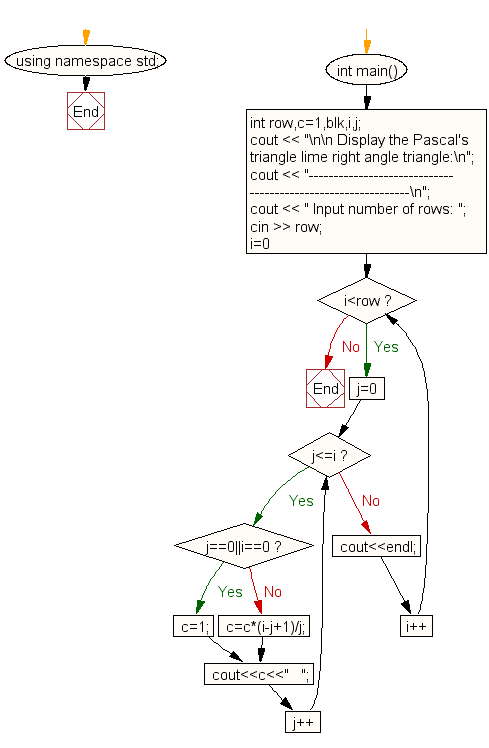﻿ C++ : Display Pascals triangle like right angle triangle

# C++ Exercises: Display Pascal's triangle like right angle triangle

## C++ For Loop: Exercise-46 with Solution

Write a C++ program to display Pascal's triangle like a right angle triangle.

Sample Solution:

C++ Code :

``````#include <iostream>
using namespace std;

int main()
{
int row,c=1,blk,i,j;
cout << "\n\n Display the Pascal's triangle lime right angle triangle:\n";
cout << "-------------------------------------------------------------\n";
cout << " Input number of rows: ";
cin >> row;
for(i=0;i<row;i++)
{
for(j=0;j<=i;j++)
{
if (j==0||i==0)
c=1;
else
c=c*(i-j+1)/j;
cout<<c<<"   ";
}
cout<<endl;
}
}
``````

Sample Output:

``` Display the Pascal's triangle lime right angle triangle:
-------------------------------------------------------------
Input number of rows: 7
1
1   1
1   2   1
1   3   3   1
1   4   6   4   1
1   5   10   10   5   1
1   6   15   20   15   6   1
```

Flowchart:C++ Code Editor: